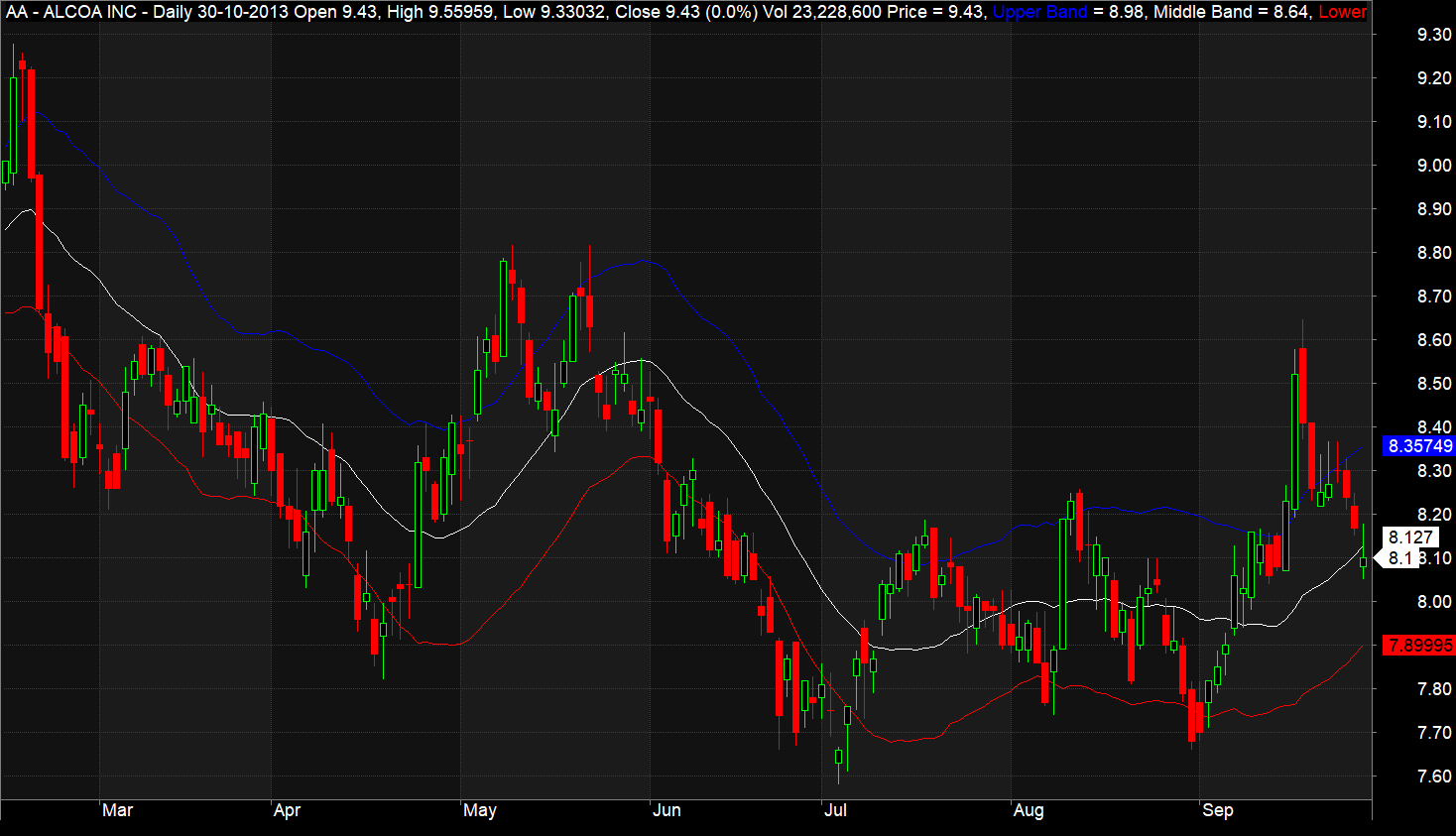Acceleration Bands are a momentum indicator that takes into account a stock’s volatility over a set period, typically 20 days or weeks. This indicator is displayed as a set of three bands on a chart: an upper band, a lower band, and a central band.

These upper and lower bands define a trading range. A breakout above or below these bands signals a swift ‘acceleration’ in the stock’s price. For example, a breakout from the upper band suggests a rapid increase in price in the coming days or weeks.

The middle band represents a simple moving average.

This tool was developed by Price Headley, the founder of bigtrends.com.

### Optimal Timeframe

Acceleration Bands are most effective in longer timeframes, such as daily or weekly charts.

### Indicator Display

The following screenshot shows Acceleration Bands on an Amibroker chart. The upper band is marked in blue, the lower band in red, and the middle band in white.```				```
//------------------------------------------------------
//
//  Formula Name:    Acceleration Bands
//  Website:         https://zerobrokerageclub.com/
//------------------------------------------------------

_SECTION_BEGIN("Acceleration Bands");

SetChartOptions(0,chartShowArrows|chartShowDates);

_N(Title = StrFormat("{{NAME}} â€“ {{INTERVAL}} {{DATE}} Open %g, Hi %g, Lo %g, Close %g (%.1f%%) {{VALUES}}", O, H, L, C, SelectedValue( ROC( C, 1 ) ) ));

Plot( Close, "Price", colorWhite, styleCandle );

LookbackPeriods = Param("LookbackPeriods",20,5,100,5);
range=(H-L)/(H+L)/2;

Upperband=MA(H*(1+2*((2*range)*100)*.01),LookbackPeriods);
Lowerband= MA(L*(1-2*((2*range)*100)*.01),LookbackPeriods);
Middleband=MA(C,LookbackPeriods);

Plot(Upperband, "Upper Band",colorBlue,styleLine);
Plot(Middleband, "Middle Band",colorWhite,styleLine);
Plot(Lowerband, "Lower Band",colorRed,styleLine);

_SECTION_END();
```
```

### Understanding the AFL Code

The AFL code for Acceleration Bands is straightforward. It calculates the upper, lower, and middle bands using these formulas:

• Upperband=MA(H*(1+2*((2*range)*100)*.01),LookbackPeriods);
• Lowerband= MA(L*(1-2*((2*range)*100)*.01),LookbackPeriods);
• Middleband=MA(C,LookbackPeriods);

where:

• MA = Simple moving average
• Range = (High – Low) / (High + Low)/2
• LookbackPeriods = Moving average time period, usually 20 days or weeks

These bands are then plotted on a candlestick chart using Amibroker’s standard “plot” function. You could also use an Exponential Moving Average (EMA) instead of a Simple Moving Average (MA) for more precise and timely breakout signals.

### Trading Rules for Long and Short Positions

To use Acceleration Bands for trading:

• Consider going long when the price crosses above the upper band and exit when it falls back below it.
• Consider going short when the price drops below the lower band and exit when it rises above it.
• Based on your risk tolerance, you could also use middle band crossovers to close open positions.### An Ultimate Guide to Using Oscillators in Technical AnalysisdexterNovember 20, 2023### The Ichimoku Trading Strategy: Amibroker AFL CodedexterOctober 24, 2023### Discover the 14-73 EMA Crossover AFLdexterSeptember 23, 2023